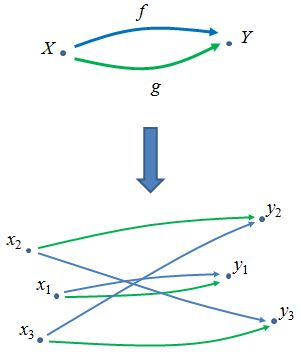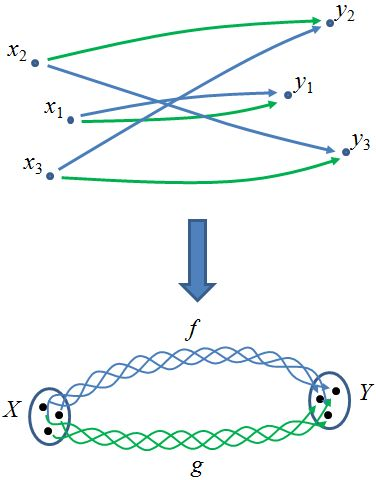Contents

category theory

# Contents

## Idea

The category of elements of a functor $F : \mathcal{C} \to$ Set is a category $el(F) \to \mathcal{C}$ sitting over the domain category $\mathcal{C}$, such that the fiber over an object $c \in \mathcal{C}$ is the set $F(c)$.

This is a special case of the Grothendieck construction, by considering sets as discrete categories.

We may think of Set as the classifying space of “Set-bundles;” see generalized universal bundle. The category of elements of $F$ is, in this sense, the Set-bundle classified by $F$. It comes equipped with a projection to $\mathcal{C}$ which is a discrete opfibration, and provides an equivalence between $\mathbf{Set}$-valued functors and discrete opfibrations. (There is a dual category of elements that applies to contravariant $\mathbf{Set}$-valued functors, i.e. presheaves, and produces discrete fibrations.)

Forming a category of elements can be thought of as “unpacking” a concrete category. For example, consider a concrete category $\mathcal{C}$ consisting of two objects $X,Y$ and two non-trivial morphisms $f,g$The individual elements of $X,Y$ are “unpacked” and become objects of the new category. The “unpacked” morphisms are inherited in the obvious way from morphisms of $\mathcal{C}$.

Note that an “unpacked” category of elements can be “repackaged”.The generalization of the category of elements for functors landing in Cat, rather than just $\mathbf{Set}$, is called the Grothendieck construction.

## Definition

Given a functor $F:\mathcal{C}\to\mathbf{Set}$, the category of elements $el(F)$ or $El_F(\mathcal{C})$ (or obvious variations) may be understood in any of these equivalent ways:

• It is the category whose objects are pairs $(c,x)$ where $c$ is an object in $\mathcal{C}$ and $x$ is an element in $F(c)$ and morphisms $(c,x)\to(c',x')$ are morphisms $u:c\to c'$ such that $F(u)(x) = x'$.

• It is the pullback along $F$ of the universal Set-bundle $U : \mathbf{Set}_* \to \mathbf{Set}$

where $U$ is the forgetful functor from pointed sets to sets.

• It is the comma category $(*/F)$, where $*$ is the inclusion of the one-point set $*:*\to \mathbf{Set}$ and $F:\mathcal{C}\to \mathbf{Set}$ is itself:

• Its opposite is the comma category $(Y/F)$, where $Y$ is the Yoneda embedding $\mathcal{C}^{op}\to [\mathcal{C},\mathbf{Set}]$ and $F$ is the functor $*\to [\mathcal{C},\mathbf{Set}]$ which picks out $F$ itself:

$El_F(\mathcal{C})$ is also often written with coend notation as $\int^\mathcal{C} F$, $\int^{c: \mathcal{C}} F(c)$, or $\int^c F(c)$. This suggests the fact the set of objects of the category of elements is the disjoint union (sum) of all of the sets $F(c)$.

• It is the (strict) oplax colimit of the composite $\mathcal{C} \xrightarrow{F} \mathbf{Set} \xrightarrow{disc} \mathbf{Cat}$; see Grothendieck construction.

When $\mathcal{C}$ is a concrete category and the functor $F:\mathcal{C}\to \mathbf{Set}$ is simply the forgetful functor, we can define a functor

$Explode(-) := El_F(-).$

This is intended to illustrate the concept that constructing a category of elements is like “unpacking” or “exploding” a category into its elements.

## Properties

The category of elements defines a functor $el : \mathbf{Set}^{\mathcal{C}} \to \mathbf{Cat}$. This is perhaps most obvious when viewing it as an oplax colimit. Furthermore we have:

###### Theorem

The functor $el : \mathbf{Set}^{\mathcal{C}} \to \mathbf{Cat}$ is cocontinuous.

###### Proof

As remarked above, $el$ is a strict weighted 2-colimit, hence we have an isomorphism

$el(F) \cong \int^{c\in \mathcal{C}} J(c) \times disc(F(c))$

where the weight $J:\mathcal{C}^{op} \to \mathbf{Cat}$ is the functor $c\mapsto c/\mathcal{C}$, and $disc:\mathbf{Set}\hookrightarrow \mathbf{Cat}$ is the inclusion of the discrete categories. But since $disc$ (regarded purely as a 1-functor) has a right adjoint (the functor which sends a -small- category $\mathcal{C}$ into its set of elements $\mathcal{C}_0$), it preserves (1-categorical) colimits. Since colimits also commute with colimits, the composite operation $\el$ also preserves colimits.

###### Theorem

The functor $el\colon \mathbf{Set}^{\mathcal{C}} \to \mathbf{Cat}$ has a right adjoint (which is maybe a more direct way to see that it is cocontinuous).

###### Proof

By a simple coend computation

$\begin{array}{rl} \mathbf{Cat}(el(F),D)&\cong \mathbf{Cat}\Big( \int^c J c\times\delta(F c), D\Big)\\ &\cong \int_c\mathbf{Cat}\big(J c\times \delta(F c),D\big)\\ &\cong \int_c \mathbf{Set}\big(F c,[J c,D]_0\big)\\ &\cong \mathbf{Set}^{\mathcal{C}}(F, K(D)) \end{array}$

where $K(D)\colon c\mapsto [J c,D]_0$.

Now for any $\mathcal{C}$, the terminal object of $\mathbf{Set}^\mathcal{C}$ is the functor $\Delta 1$ constant at the point. The category of elements of $\Delta 1$ is easily seen to be just $\mathcal{C}$ itself, so the unique transformation $F\to \Delta 1$ induces a projection functor $\pi_F: \el(F) \to \mathcal{C}$ defined by $(c,x)\mapsto c$ and $u\mapsto u$. The projection functor is a discrete opfibration, and can be viewed also as a $\mathcal{C}$-indexed family of sets. When we regard $\el(F)$ as equipped with $\pi_F$, we have an embedding of $\mathbf{Set}^\mathcal{C}$ into $\mathbf{Cat}/\mathcal{C}$.

Note that the canonical projection $\operatorname{El}(F) \to \mathbf{C}$ is not usually full. For example, let $\mathbf{B}\mathbb{N}$ be the one-object category which carries the monoid $(\mathbb{N}, +)$ as its endomorphism monoid, and let $F$ be the action of $(\mathbb{N}, +)$ on the set $\mathbb{N}$ by $n.m = m + n$. Then the image of any hom-set between $k, k'$ is a singleton subset of $\mathbb{N}$.

More generally, the universal covering groupoid of a groupoid is just the category of elements of its action on itself by composition. Since this action is faithful and transitive, hom-sets in the category of elements are always $0$ or $1$, while objects in the groupoid might have nontrivial automorphism groups.

## Examples

### Representable Presheaves

Let $Y(c):\mathcal{C}^{op}\to \mathbf{Set}$ be a representable presheaf with $Y(c)(d)=Hom_{\mathcal{C}}(d,c)$. Consider the contravariant category of elements $\int_\mathcal{C} Y(c)$ . This has objects $(d_1,p_1)$ with $p_1\in Y(c)(d_1)$, hence $p_1$ is just an arrow $d_1\to c$ in $\mathcal{C}$. A map from $(d_1, p_1)$ to $(d_2, p_2)$ is just a map $u:d_1\to d_2$ such that $p_2\circ u =p_1$ but this is just a morphism from $p_1$ to $p_2$ in the slice category $\mathcal{C}/c$. Accordingly we see that $\int_\mathcal{C} Y(c)\simeq \mathcal{C}/c$ .

This equivalence comes in handy when one wants to compute slices of presheaf toposes over representable presheaves $Y(c)$ since $PSh(\int_\mathcal{C} F) \simeq PSh(\mathcal{C})/F$ in general for presheaves $F:\mathcal{C}^{op}\to \mathbf{Set}$ , whence $PSh(\mathcal{C})/Y(c) \simeq PSh(\mathcal{C}/c)$ . An instructive example of this construction is spelled out in detail at hypergraph.

### Action Groupoid

In the case that $\mathcal{C} = \mathbf{B}G$ is the delooping groupoid of a group $G$, a functor $\varrho : \mathbf{B}G \to \mathbf{Set}$ is a permutation representation $X$ of $G$ and its category of elements is the corresponding action groupoid $X/\!/G$.

###### Proof

This is easily seen in terms of the characterization $el(\varrho)\cong (*/\varrho)$, the category having as objects triples $(*,*; *\to \varrho(*)=X)$, namely elements of the set $X=\varrho(*)$, and as arrows $x\to y$ those $g\in \mathbf{B}G$ such that

commutes, namely $g . x=\varrho(g)(x)=y$. We can also present the right adjoint to $el(-)$: one must consider the functor $J\colon \mathbf{B}G^{op}\to \mathbf{Cat}$, which represents $G$ in $\mathbf{Cat}$, and sends the unique object $*\in \mathbf{B}G$ to $*/\mathbf{B}G\cong G/\!/G$, the left action groupoid of $G$. The functor $J$ sends $h\in G$ to an automorphism of $G/\!/G$, obtained multiplying on the right $x\to g x$ to $x h\to x g h$.

Now for any category $D$, $K( D)(*)$ is exactly the set of functors $[G/\!/G, D]$, which inherits from $G/\!/G$ an obvious action: given $F\in [G/\!/G, D]$ we define $F^h=J(h)^*F=F \circ J(h) \colon g \mapsto F(g h)$.

### Category of simplices

For a simplicial set regarded as a presheaf on the simplex category, the corresponding category of elements is called its category of simplices. See there for more.

## Reference

A very nice introduction emphasizing the connections to monoid theory is ch. 12 of

Last revised on September 8, 2023 at 16:01:20. See the history of this page for a list of all contributions to it.